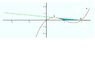# Integration, area under line and curve.

tweety1234

## Homework Statement

The diagram shows the graph of the curve C whose equation is $$y = x(x-1)(x-2)$$

a) find the equation of the tangent at the pont P whose x coordinate is $$\frac{1}{2}$$

b) show that the point Q(2,0) lies on the tnagent and on C.

the equation of the tangent line is $$y = -\frac{1}{4}x +\frac{1}{2}$$

But I am not sure how to work out the shaded area?

Is it the line minus the x-axis?

$$\int_\frac{1}{2}^2 -\frac{1}{4}x + \frac{1}{2} -( \frac{3}{2})$$ $$dx$$

?

https://www.physicsforums.com/attachment.php?attachmentid=18690&d=1241015314

#### Attachments

•Untitled.jpg
11.6 KB · Views: 448
Last edited:

Staff Emeritus
Gold Member
Draw rectangles of differential area. What curve does the top of the rectangle touch? What curve does the bottom touch? Hint: For the latter question the answer is different on $[\frac{1}{2},1)$ than it is on $(1,2]$.

tweety1234
Draw rectangles of differential area. What curve does the top of the rectangle touch? What curve does the bottom touch? Hint: For the latter question the answer is different on $[\frac{1}{2},1)$ than it is on $(1,2]$.

The height of the rectanlge touches the tangent line, and the bottom touches the x-axis?

Why are you also including the area under the x-axis , which is not shaded?

Last edited:
Chewy0087
Basically what it wants you to do here is like you said work out the area of the triangle PQY. Then use definite integration between P & 1 (when the curve goes below). I don't think you need the coordinates of Q at all here.

Can you follow it from there?

Last edited:
tweety1234
Basically what it wants you to do here is like you said work out the area of the triangle PQY. Then use definite integration between P & 1 (when the curve goes below). I don't think you need the coordinates of Q at all here.

Can you follow it from there?

Edit : I just realized you may not have worked out P yet; if you haven't here's a tip :differentiating to work out turning points.

Do you mean 'PQX'?

Chewy0087
sorry, yes =p x being ( 0.5 , 0 ) :D

Last edited:
tweety1234
sorry, yes =p

I don't think that makes a triangle though.

Chewy0087
Hm? My fault, perhaps I'm not being very clear.

You take P right? Draw a line straight down to the X-axis, then follow it across to Q. This will form a right angled triangle. I think you can follow my train of thought here, if you can work out the area of that triangle...think about how you can work out the area of the shaded region using definite integration!

tip;
$$\int ^{1} _{0.5}$$

=D

tweety1234
Hm? My fault, perhaps I'm not bieng very clear.

You take P right? Draw a line straight down to the X-axis, then follow it across to Q. This will form a right angled triangle.

then definiatley integrate for

$$\int ^{1} _{0.5}$$

Oh right,

so your saying find the area of that triangle then find the area under the curve using definite integration from 0.5 to 1 and minus this area away from the triangle ?

Chewy0087
bingo

tweety1234
Okay I'll try that and see what I get!

thanks.